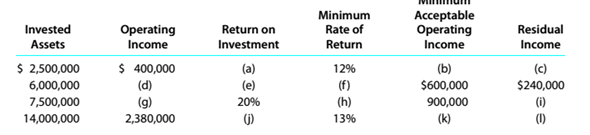Chapter 14, Problem 14.15E

Chapter
Section
Textbook Problem

Determining missing items in return on investment and residual income computations The following table presents various rates of return on investment and residual incomes:Determine the missing items, identifying each item by the appropriate letter.

To determine

Concept Introduction:

Rate of return on investment represents the investment performance. It is beneficial for investor to compare different investments and select best investment. The benefit or return from the original cost of investment is return on investment.

The missing items in return on investment and residual income computations.

Explanation

Calculation of rate of return on investment:

Rate of return on investment = Income from operation Invested assets =$400,000$2,500,000=16%

Calculation of acceptable return:

Minimum acceptable return = $2,500,000×0.12=$300,000

Calculation of residual income:

Residualincome = $400,000 -$300,000= $100,000 Calculation of income from operation: Income from operation= Minimum acceptable income+Residualincome$ 600,000+$240,000=$ 840,000

Calculation of rate of return on investment:

Rate of return on investment = $840,000$6,000,000=14%

Calculation of minimum rate of return:

Minimum rate of return = $600,000$6,000,000=10%

Calculation of income from operation:

Income from operation = \$7,500,000×0

Still sussing out bartleby?

Check out a sample textbook solution.

See a sample solution

The Solution to Your Study Problems

Bartleby provides explanations to thousands of textbook problems written by our experts, many with advanced degrees!

Get Started

How do Accounts Payable and Accounts Receivable differ?

College Accounting (Book Only): A Career Approach

Should an economic model describe reality exactly?

Essentials of Economics (MindTap Course List)

PAYBACK PERIOD Refer to problem 11-1. What is the projects payback?

Fundamentals of Financial Management (MindTap Course List)

What are some actions that stockholders can take to ensure that managements and stockholders interests are alig...

Fundamentals of Financial Management, Concise Edition (with Thomson ONE - Business School Edition, 1 term (6 months) Printed Access Card) (MindTap Course List)